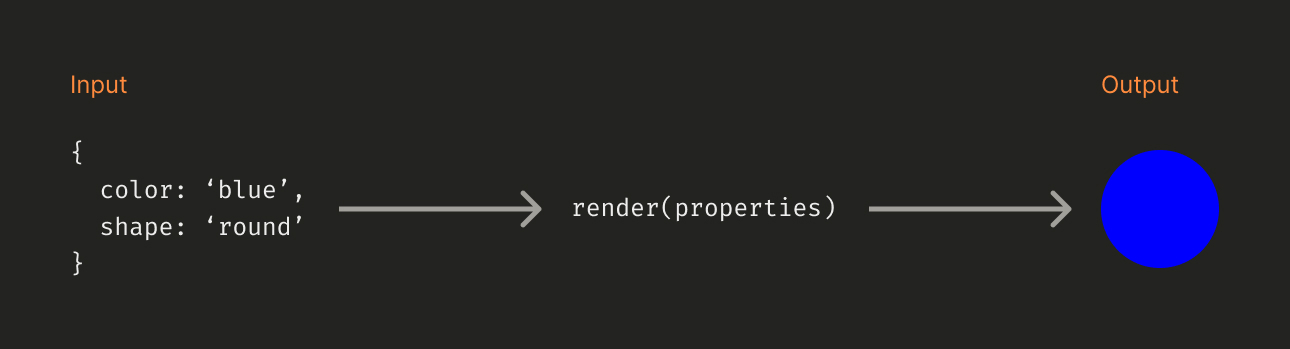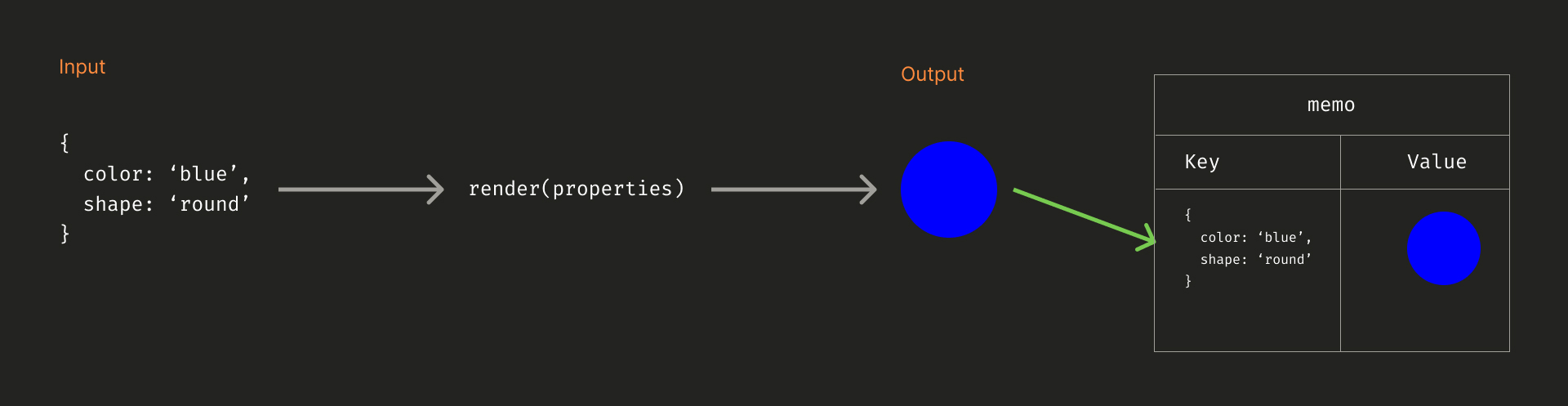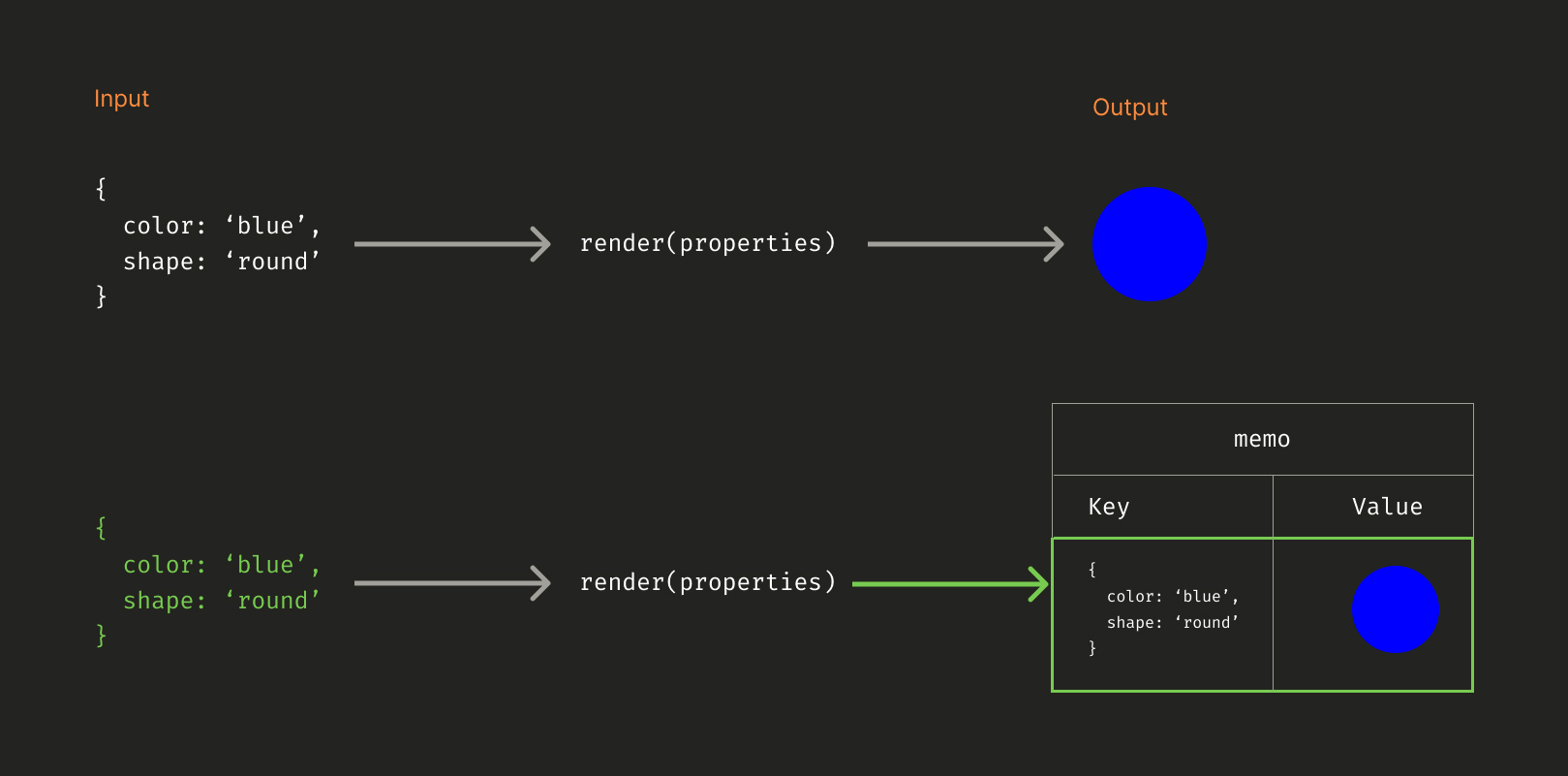Memoization is a code optimization technique that saves a processing result in memory so that we skip the computation and return the same result given the same input later.

To illustrate that, let’s say we have an expensive function called `render`. The `render` function takes some properties as an argument and renders a 2D model based on the properties we passed.Due to the costly nature of the `render` function, we don’t want to do the same calculation again with the same input. So at each render, we store the input and the output in memory as key-value pairs.It allows us a quick lookup the next time we come across the same input saving us computing time.## Memoization in JavaScript

Now let’s see how we could implement memoization in javaScript. Here we have a simple function that adds two to the number we pass and return the result:

``````function addTwo(num) {
return num + 2;
}``````

What we want to do is to create a memoized version of that function. To achieve that, let’s define the `memoize` function that takes as a parameter the function we want to memoize and returns a new function:

``````function memoize(fn) {
const memo = {};
return (...args) => {
const argsStr = JSON.stringify(args);

if (memo[argsStr]) {
const result = memo[argsStr];
return result;
}

const result = fn(...args);
memo[argsStr] = result;
return result;
};
}``````

We defined the `memo` variable as a closure to map the inputs to their respective outputs. The new function we return next contained all the memoization logic. If we already came across a given argument, we return the corresponding result from `memo`:

``````function memoize(fn) {
//...
return (...args) => {
if (memo[argsStr]) {
return memo[argsStr];
}
//...
};
}``````

Otherwise, we proceed to the computation and save the result in `memo`:

``````function memoize(fn) {
//...
return (...args) => {
//...
const result = fn(...args);
memo[argsStr] = result;
return result;
};
}``````

Now, we’re all set to create the memoized version of the `addTwo` function:

``````const memoizedAddTwo = memoize(addTwo);

console.log(memoizedAddTwo(2)); //==> 4 known input. It uses memo!

## When to use memoization

In the real world, it’s overkill to use memoization for functions like `addTwo`. Instead, we use it when a process is time-consuming.

## The issue with memoization

Memoization saves us time for the price of memory space. Used too frequently, the memory space we use to map each input and output grows proportionally. We can reduce that impact by deleting a mapping each time we return the result from a known input to free up some space.

``````//...
if (memo[argsStr]) {
const result = memo[argsStr];
delete memo[argsStr];
return result;
}
//...``````

## Wrap up

Memoization allows us to keep track of the different results of an expensive function so that, on known inputs, we don’t have to repeat the calculation. However, this technique trades time for memory space, so we must use it carefully.